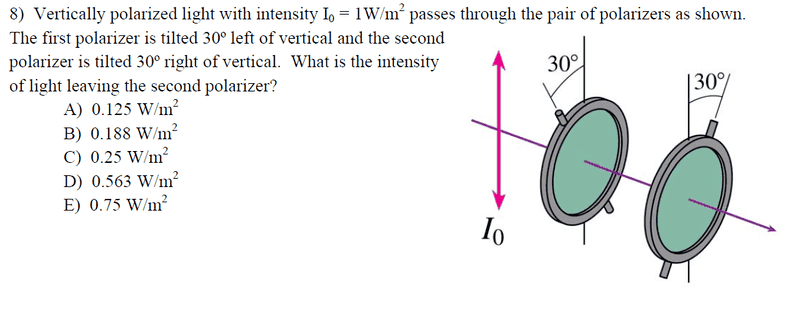So I know the equation:

Itrans = I0 Cos2θ

Since this light starts out as polarized, there is no need to cut it in half like you do for unpolarized light. So I did the equation through the first polzarizer, using 30 degree as my angle, and came out with I=0.75

Now I used that 0.75 as my new I0 as the light went through the 2nd polarizer, this time I used 60 degrees as my θ and I got 0.1875, which would be B.

However, I saw the same problem elsewhere where the guy got D) as the answer because he used 30 for θ instead of 60.

blue_leaf77
Homework Helper
I agree with you.

edit: I didnt notice the opposite axis of the second one, I would say you are correct in your first assumption and I agree with the rest of the posters. use 30 then 60

Last edited:
Since you have 2 polarized sheets at 30 degree I would use 1(cos30^2)^2

That gives 0.5625, can you explain WHY you would use 30 degree for the second polarizer when the axis is 60 after the coming out of the first one?

Anyone else want to chime in, I still don't know which is correct.

TSny
Homework Helper
Gold Member
Anyone else want to chime in, I still don't know which is correct.
B is the right answer. Your analysis of using 30 then 60 degrees is correct.

B is the right answer. Your analysis of using 30 then 60 degrees is correct.
Thank you